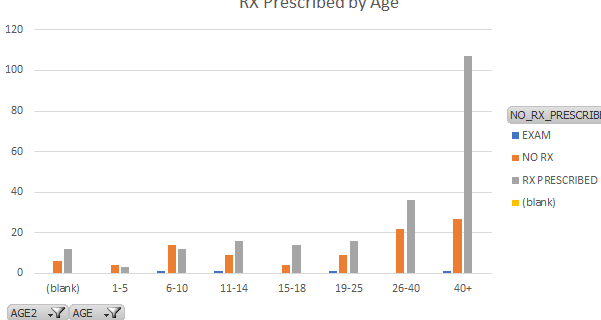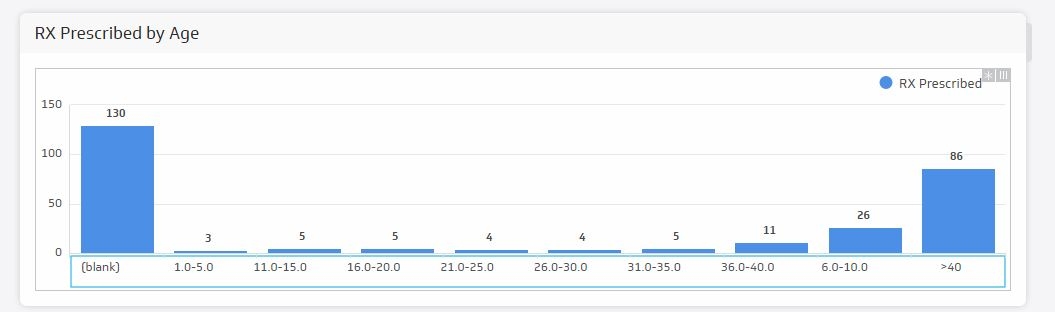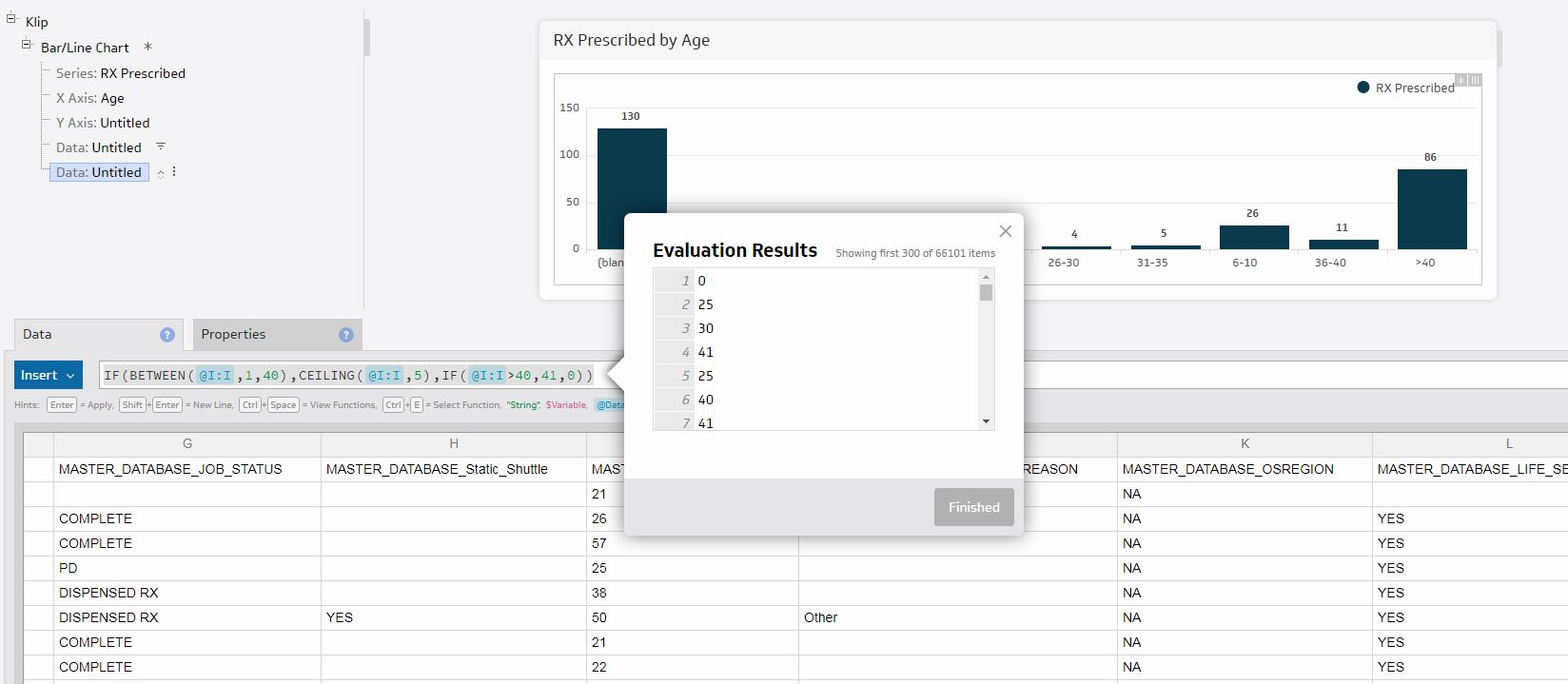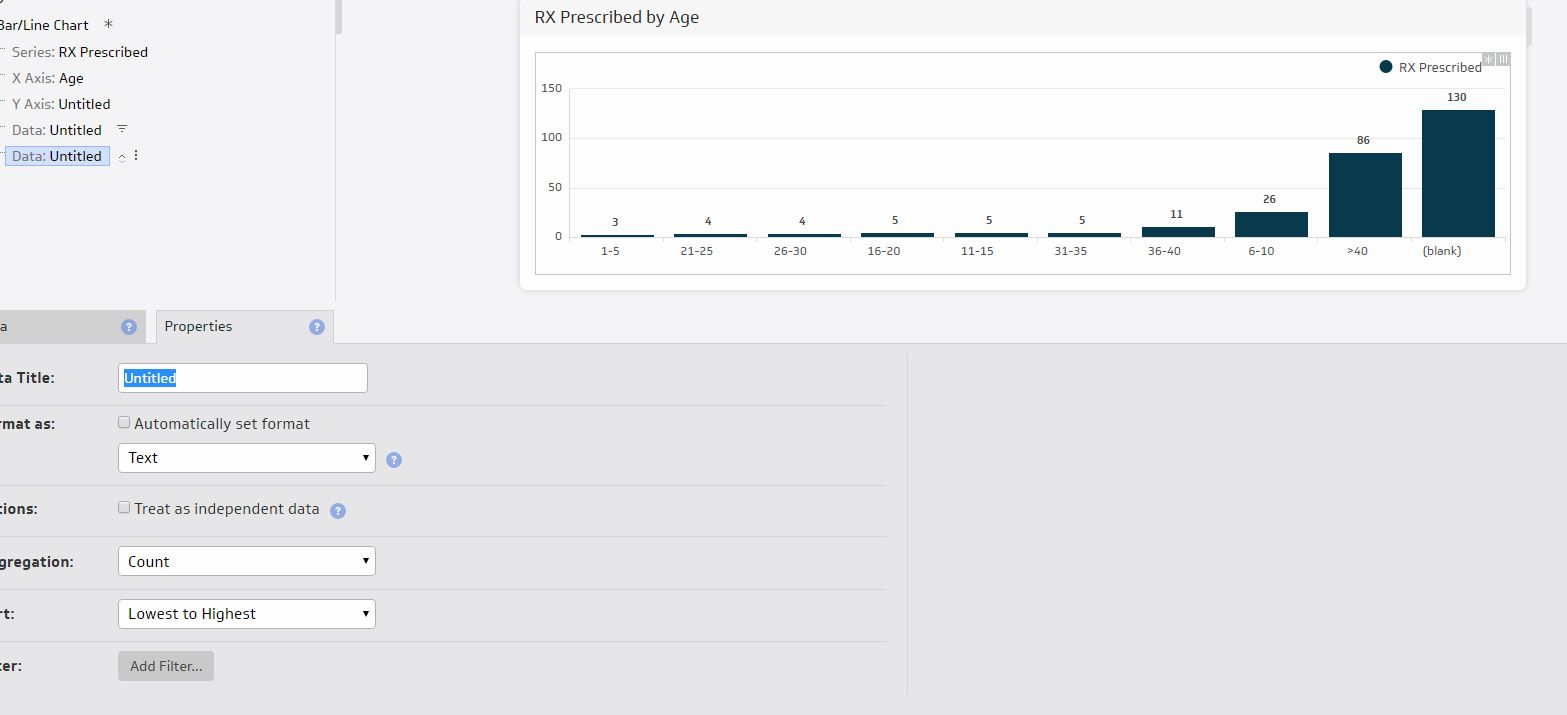Hi ,

I have data containing ages in which I am trying to group in to custom age ranges. It would appear like the screenshot below. How do I go about this in building a klip ?Thanks,

Kevin

•Janice Janczyn

Hi Kevin,

This type of grouping is simplest when each range covers the same number of ages because then you can use a single value to group most of the data using the CEILING function. For example, if you want your X Axis to have be groups of 5 year ranges, that is, 1-5,6-10, 11-15....36-40 as well as (blank) and >40, you could build it using something like the following:

IF( BETWEEN( data, 1, 40),
CONCAT( CEILING( data, 5)  - 4, "-", CEILING( data, 5 ) ),
IF( data > 40, ">40", "(blank)" ) )

If your Series is a count of the number of items in each age range, use the following to

IF( BETWEEN( data, 1, 40 ),
CEILING( data, 5 ) ,
IF( data > 40, ">40", "(blank)" ) )

On the X Axis Properties tab, set the format to text and select Group repeating labels. Then on the Series Properties tab, set Aggregation to Count.

If you want your age ranges to be more variable, you will either have to build your formula to handle the special cases or pre-process the data so that it contains the age ranges you need.

I hope this helps!

Janice

•Kevin Burke

Hi Janice,

I was curious if you know of a way to have the age ranges sorted from lowest to highest?

Because it's formatted as text , the sorting is not in the correct order

Thanks so much !•Janice Janczyn

Hi Kevin,

Yes, but first, to eliminate the decimal points in your x-axis, wrap the NUMBERFORMAT function around your each number manipulation result. By default this will set the result to use 0 decimal places. For example:

CONCAT( NUMBERFORMAT( CEILING( data, 5)   - 4 ), "-", NUMBERFORMAT( CEILING( data, 5 ) ) )

To sort numerically, add hidden data with basically the same formula as the x-axis, but formulated to return numeric results, so 41 instead of ">40" and 0 instead of "blank". The CEILING formula is simplified to return only 1 numeric value.

IF( BETWEEN( data, 1, 40),
CEILING( data, 5)
IF( data > 40,
41,
0 )  )

Then Sort this hidden data from lowest to highest and your klip will now be ordered correctly.

Thanks,
Janice

•Kevin Burke

Thanks ! The decimal point formula worked.

It seems the sort is still not working - here is my formula and even if I try formatting as Text or Number and trying Count or Sum aggregation they don't want to sort properly•Janice Janczyn

Hi Kevin,

Please enable support access and email support@klipfolio.com so we can take a closer look.

Thank you,
Janice

•Kevin Burke

Support access enabled

Thank you

•Janice Janczyn

Hi Kevin,

I've created a support ticket for you. Please let us know which dashboard and klip to look at.

Thank you,
Janice